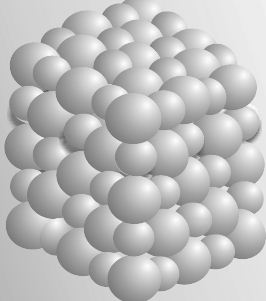# Define and Describe on Formula Mass

Some substances such as sodium chloride do not contain discrete molecules as their constituent units. In such compounds, positive (sodium) and negative (chloride) entities are arranged in a three-dimensional structure, as shown in Figure.Fig: Packing of Na and Cl ions in sodium chloride

It may be noted that in sodium chloride, one Na is surrounded by six CI and vice versa.

The formula such as NaCl is used to calculate the formula mass instead of molecular mass as in the solid state sodium chloride does not exist as a single entity.

Thus, formula mass of sodium chloride = atomic mass of sodium + atomic mass of chlorine

= 23.0 μ + 35.5 μ = 58.5 μ